## 6.2.2 Bulk Case

By neglecting in the six moments transport model (eqns. (2.178) to (2.183)) all terms containing derivatives, equations for the homogeneous case are obtained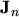(6.4)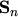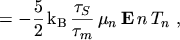(6.5)

 0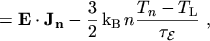(6.6) 0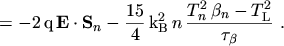(6.7)

Inserting eqn. (6.4) into eqn. (6.6) and eqn. (6.5) into eqn. (6.7) yields the equations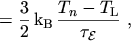(6.8)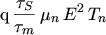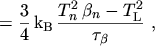(6.9)

which can further be reduced to a bulk relation for the non-MAXWELLian parameter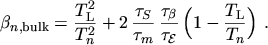(6.10)

Unfortunately, the models for the relaxation times are not accurate enough to obtain a realistic estimate for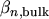. Therefore the following fit to Monte Carlo data has been developed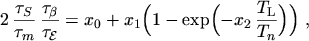(6.11)

with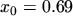,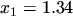, and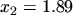. This expression is accurate for doping concentrations aroundbut the doping dependence ofis only relevant at lower temperatures (Fig. 6.3).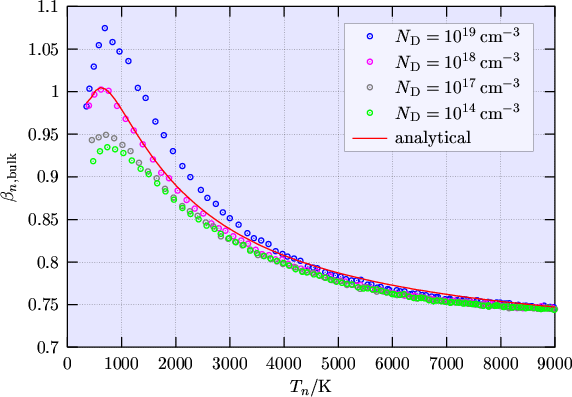However, the presented formulation fordid not solve the problem of anomalous SOI simulation results--presumably because the decrease of the kurtosis with increasing temperature occurs too slowly.

M. Gritsch: Numerical Modeling of Silicon-on-Insulator MOSFETs PDF